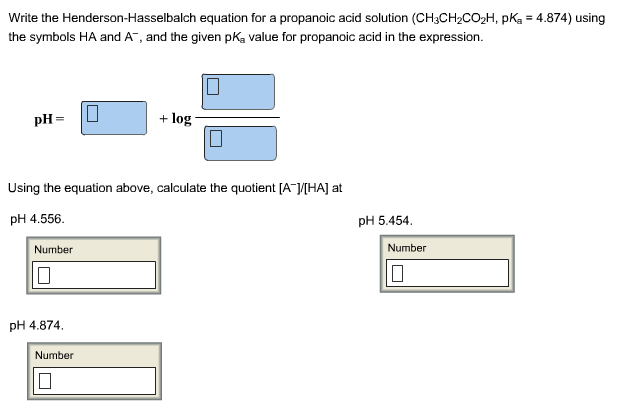# Problem: Write the Henderson-Hasselbalch equation for a propanoic acid solution (CH3CH2CO2H, pKa = 4.874) using the symbols HA and A-, and the given pKa value for propanoic acid in the expression. Using the equation above, calculate the quotient [A-]/[HA] at pH           (a) 4.556          (b) 5.454          (c) pH 4.874

###### FREE Expert Solution
86% (482 ratings)###### Problem Details

Write the Henderson-Hasselbalch equation for a propanoic acid solution (CH3CH2CO2H, pKa = 4.874) using the symbols HA and A-, and the given pKa value for propanoic acid in the expression.

Using the equation above, calculate the quotient [A-]/[HA] at pH

(a) 4.556

(b) 5.454

(c) pH 4.874